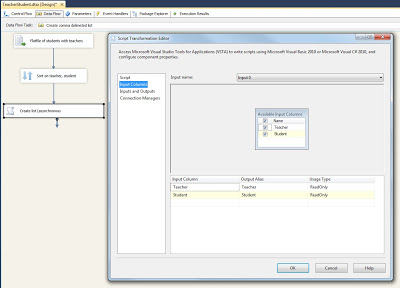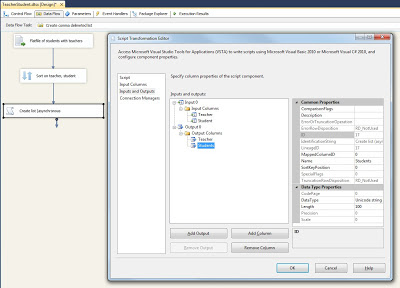# Creating a comma separated list of related records

Case
I have a list of teacher and student combinations with one combination per record and I want to created a comma delimited list of students per teacher.Solution
If your source is a database then the easiest solution is a TSQL query like this:
`--TSQL QueryWITH UniqueTeachers AS( SELECT  DISTINCT Teacher FROM  TeacherStudentTable)SELECT  Teacher,   Students = STUFF((      SELECT  ',' + Student      FROM  TeacherStudentTable      WHERE  Teacher = UniqueTeachers.Teacher      ORDER BY Student      FOR XML PATH(''), TYPE).value('.','varchar(100)'), 1, 1, '')FROM  UniqueTeachersORDER BY Teacher`The query in SSIS as source

If your source is for example a flat file or a database that doesn't support a query like this, then there are also options within SSIS. For this solution I use a asynchronous Script Component.

1) Sorted source
We need a sorted source because we are comparing records with each other. In this case make sure the source is sorted on teacher first (and optional secondly on student).Add Sort transformation if source isn't sorted

2) Script Component
Add a Script Component (type transformation) and select the Teacher and Student columns as ReadOnly input columns.Input columns: Teacher and Student

3) Asynchronous
We need to make the Script Component asynchronous because it throws out a different number of rows than there are incomming. Go to the Inputs and Outputs pane, click on Output 0 and change the SynchronousInputID to None.Asynchonous

4) Output
We now need to create an output for the Script Component. Expand the Output 0 and add two columns:
Teacher (same data type and size as the input column teacher)
Students (same data type as the input column student, but larger to fit multiple student names)Output columns

5) The Script
Copy the three variables and the two methods to your Script Component (and remove any other existing methods).
`// C# Codeusing System;using System.Data;using Microsoft.SqlServer.Dts.Pipeline.Wrapper;using Microsoft.SqlServer.Dts.Runtime.Wrapper;[Microsoft.SqlServer.Dts.Pipeline.SSISScriptComponentEntryPointAttribute]public class ScriptMain : UserComponent{    bool initialRow = true;     // Indicater for the first row    string teacher = "";        // Name of the teacher to track teacherchanges between rows    string students = "";       // The comma delimited list of students    public override void Input0_ProcessInput(Input0Buffer Buffer)    {        // Loop through buffer        while (Buffer.NextRow())        {            // Process an input row            Input0_ProcessInputRow(Buffer);            // Change the indicator after the first row has been processed            initialRow = false;        }                // Check if this is the last row        if (Buffer.EndOfRowset())        {            // Fill the columns of the existing output row with values            // from the variable before closing this Script Component            Output0Buffer.Teacher = teacher;            Output0Buffer.Students = students;        }    }    public override void Input0_ProcessInputRow(Input0Buffer Row)    {        if (initialRow)        {            // This is for the first input row only                        // Create a new output row            Output0Buffer.AddRow();            // Now fill the variables with the values from the input row            teacher = Row.Teacher;            students = Row.Student;        }        else if ((!initialRow) & (teacher != Row.Teacher))        {            // This isn't the first row, but the teacher did change            // Fill the columns of the existing output row with values            // from the variable before creating a new output row            Output0Buffer.Teacher = teacher;            Output0Buffer.Students = students;            // Create a new output row            Output0Buffer.AddRow();            // Now fill the variables with the values from the input row            teacher = Row.Teacher;            students = Row.Student;        }        else if ((!initialRow) & (teacher == Row.Teacher))        {            // This isn't the first row, and the teacher did not change            // Concatenate the studentsname to the variable            students += "," + Row.Student;        }    }    // Little explanation:    // Rows are created in memory with .AddRow()    // and will be submitted to the output when a    // new / subsequent row is created or when    // the last buffer has been finished.}`

or in VB.Net

`'VB.Net codeImports SystemImports System.DataImports System.MathImports Microsoft.SqlServer.Dts.Pipeline.WrapperImports Microsoft.SqlServer.Dts.Runtime.Wrapper<Microsoft.SqlServer.Dts.Pipeline.SSISScriptComponentEntryPointAttribute()> _<CLSCompliant(False)> _Public Class ScriptMain    Inherits UserComponent    Private initialRow As Boolean = True    ' Indicater for the first row    Private teacher As String = ""          ' Name of the teacher to track teacherchanges between rows    Private students As String = ""         ' The comma delimited list of students    Public Overrides Sub Input0_ProcessInput(Buffer As Input0Buffer)        ' Loop through buffer        While Buffer.NextRow()            ' Process an input row            Input0_ProcessInputRow(Buffer)            ' Change the indicator after the first row has been processed            initialRow = False        End While        ' Check if this is the last row        If Buffer.EndOfRowset() Then            ' Fill the columns of the existing output row with values            ' from the variable before closing this Script Component            Output0Buffer.Teacher = teacher            Output0Buffer.Students = students        End If    End Sub    Public Overrides Sub Input0_ProcessInputRow(Row As Input0Buffer)        If initialRow Then            ' This is for the first input row only            ' Create a new output row            Output0Buffer.AddRow()            ' Now fill the variables with the values from the input row            teacher = Row.Teacher            students = Row.Student        ElseIf (Not initialRow) And (teacher <> Row.Teacher) Then            ' This isn't the first row, but the teacher did change            ' Fill the columns of the existing output row with values            ' from the variable before creating a new output row            Output0Buffer.Teacher = teacher            Output0Buffer.Students = students            ' Create a new output row            Output0Buffer.AddRow()            ' Now fill the variables with the values from the input row            teacher = Row.Teacher            students = Row.Student        ElseIf (Not initialRow) And (teacher = Row.Teacher) Then            ' This isn't the first row, and the teacher did not change            ' Concatenate the studentsname to the variable            students += "," & Convert.ToString(Row.Student)        End If    End Sub    ' Little explanation:    ' Rows are created in memory with .AddRow()    ' and will be submitted to the output when a    ' new / subsequent row is created or when    ' the last buffer has been finished.End Class`

Note: You can change the delimiter in C# line 70 and VB.Net line 64.

6) Testing
For testing purposes I added a derived column and a couple of data viewer.The result

﻿

Conclusion: both methods have the same result. For a SQL database source, the T-SQL method is probably a little faster. If you can do the sort in the source the performance differences will diminish.

In one of my next blog posts I will show you how to split a comma separated list in to records.